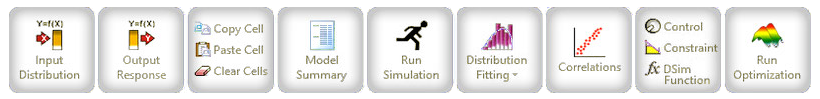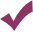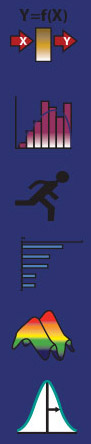Include Top

DiscoverSim - Discovery through Simulation

Click the image below to navigate through DiscoverSim's virtual menu.Why choose DiscoverSim ?

•Excel add-in for Monte Carlo Simulation and Optimization

•Easy to use with Six Sigma language - specify Inputs (X's) and Outputs (Y's). Ideal for DFSS, Risk and Project Management.

•Powerful - 53 continuous and 10 discrete distributions. Distribution Fitting with Automatic Best Fit, specify correlations between inputs.

•Fast - accelerated calculations with Excel spreadsheet interpreter.

•Identify important X's with Sensitivity Analysis based on correlation or stepwise regression - includes quadratic terms!

•Discover solutions to difficult problems with Global Optimization.

•Optimize statistics such as dpm or Ppk and allow for constraints.

•DiscoverSim is also bundled with SigmaXL Version 9 for statistical and graphical analysis.DISTRIBUTIONS IN DISCOVERSIMTM

• Common Continuous
• Normal
• Triangular
• Uniform
• Pearson Family  (specify Mean, StdDev, Skewness, Kurtosis)
• Log Normal**
• Exponential**
• Weibull**
• Pert
• Custom Continuous
• Beta
• Beta (4 Parameter)
• Box-Cox**
• Burr**
• Cauchy
• Chi-Squared**
• Chi-Squared with Scale  (3 Parameter)**
• Error Function (ERF)
• F**
• F with Sacle  (4 Parameter)**
• Fatigue Life**
• Fisk**
• Folded Normal
• Frechet**
• Gamma**
• Generalized Error
• Generalized Gamma**
• Generalized Logistic
• Generalized Pareto
• Half Normal**
• Inverse Gama**
• Inverse Gaussian
• Johnson SB
• Johnson SL
• Johnson SU
• Laplace
• Largest Extreme Value
• Levy
• Logistic
• Log Gamma**
• Log Logistic**
• Maxwell
• Non-Central Chi-Squared**
• Non-Central F**
• Non-Central T
• Pareto
• Power
• Rayleigh
• Reciprocal
• Skew Normal
• Smallest Extreme Value
• Student's T
• Student's T with Location and Scale (3 Parameter)
• Von Mises
• Discrete
• Bernoulli  (Yes/No)
• Binomial
• Geometric
• Hypergeometric
• Logarithmic
• Negative Binomial
• Poisson
• Step
• Uniform (Discrete)
• Custom Discrete
• SIP  (Stochastic Information Packet - Unweighted Sequential Custom Discrete)

**Distribution with and without threshold

Web Demos

Our CTO and Co-Founder, John Noguera, regularly hosts free Web Demos featuring SigmaXL and DiscoverSim